# Texas Go Math Grade 2 Lesson 4.3 Answer Key Describe Equal Parts

Refer to our Texas Go Math Grade 2 Answer Key Pdf to score good marks in the exams. Test yourself by practicing the problems from Texas Go Math Grade 2 Lesson 4.3 Answer Key Describe Equal Parts.

## Texas Go Math Grade 2 Lesson 4.3 Answer Key Describe Equal Parts

Explore

Draw to show the pattern blocks you used.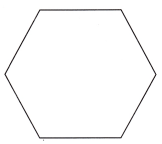Two pattern blocks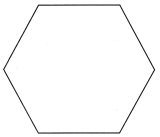six pattern blocksExplanation:
Pattern Blocks are much more than simply a set of colored blocks.
They are a very powerful mathematics manipulative that may be used to support the teaching and learning of a variety of topics like,

FOR THE TEACHER • Have children find 2 of the same pattern blocks that can fit on top of the first shape shown. Then have children draw to record the shapes. Then repeat with 6 pattern blocks.
HexagonExplanation:
The pink Hexagon is a regular hexagon  that is,
all of the sides are the same length and all of the angles are the same size (congruent).
Likewise the blue triangle is regular, that is all of the sides are the same length and the angles are congruent, so it is referred to as an equilateral triangle.

Math Talk
Mathematical Processes

Describe how equal parts for the two hexagons are different.
Hexagon has 6 sides and it can be sub divided in to 6 parts or two halves.
Explanation:as shown above hexagon pictures the two hexagons are divided two parts and six parts.

Model and Draw

The wholes are the same size. How are the sizes of the equal parts different?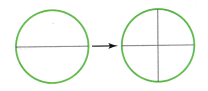There are more equal parts in the second whole.
These parts are ___________.
parts are four
Explanation:
The inverse relationship between the size of the fractional part and the number of equal parts in the whole when given the same size whole differs.
The greater the number of parts, the smaller the size of the parts and
the smaller the number of parts, the greater the size of the parts.There are fewer equal parts in the second whole.
These parts are ___________.
Parts are four
Explanation:
The inverse relationship between the size of the fractional part and the number of equal parts in the whole when given the same size whole differs.
The greater the number of parts, the smaller the size of the parts and
the smaller the number of parts, the greater the size of the parts.

Share and Show

Draw to show smaller equal parts on the second whole.

Question 1.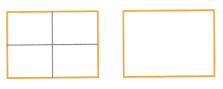Explanation:
The inverse relationship between the size of the fractional part and the number of equal parts in the whole when given the same size whole differs.
The greater the number of parts, the smaller the size of the parts and
the smaller the number of parts, the greater the size of the parts.

Question 2.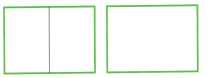Explanation:
The inverse relationship between the size of the fractional part and the number of equal parts in the whole when given the same size whole differs.
The greater the number of parts, the smaller the size of the parts and
the smaller the number of parts, the greater the size of the parts.

Draw to show larger equal parts on the second whole.

Question 3.Explanation:
The inverse relationship between the size of the fractional part and the number of equal parts in the whole when given the same size whole differs.
The greater the number of parts, the smaller the size of the parts and
the smaller the number of parts, the greater the size of the parts.

Question 4.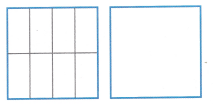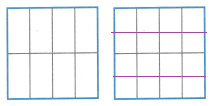Explanation:
The inverse relationship between the size of the fractional part and the number of equal parts in the whole when given the same size whole differs.
The greater the number of parts, the smaller the size of the parts and
the smaller the number of parts, the greater the size of the parts.

Problem Solving

Read each label. Draw to show the equal parts on the second whole.

Question 5.
more equal parts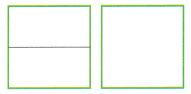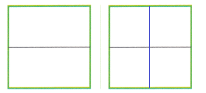Explanation:
The inverse relationship between the size of the fractional part and the number of equal parts in the whole when given the same size whole differs.
The greater the number of parts, the smaller the size of the parts and
the smaller the number of parts, the greater the size of the parts.

Question 6.
fewer equal parts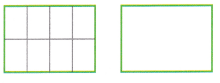Explanation:
The inverse relationship between the size of the fractional part and the number of equal parts in the whole when given the same size whole differs.
The greater the number of parts, the smaller the size of the parts and
the smaller the number of parts, the greater the size of the parts.

Question 7.
H.O.T. Two posters are the same size. A half of one poster is red, and a fourth of the other poster is blue.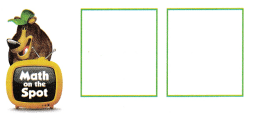Is the red part or the blue part larger? Draw and write to explain.
Red part is larger.Explanation:
The inverse relationship between the size of the fractional part and the number of equal parts in the whole when given the same size whole differs.
The greater the number of parts, the smaller the size of the parts and
the smaller the number of parts, the greater the size of the parts.

Question 8.
H.O.T. Multi-Step Three cakes are the same size. Read each label. Draw to show the cakes divided into those equal parts.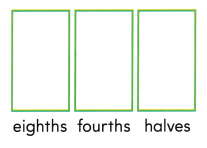How do the sizes of the parts change when there are fewer equal parts?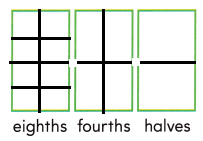Explanation:
The inverse relationship between the size of the fractional part and the number of equal parts in the whole when given the same size whole differs.
The greater the number of parts, the smaller the size of the parts and
the smaller the number of parts, the greater the size of the parts.

Question 9.
Analyze Two cards are the same size. The red card is cut into halves. The green card is cut into fourths. Which card has more parts?
the ___________ card
Suppose there is a blue card that is the same size as the green card. If the blue card is cut into more equal parts than the green card, will the blue parts be larger? Explain.
Blue card has more parts.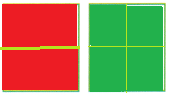In the above picture, the red card is cut into halves and the  green card is cut into fourths.
The green card has more parts.
Explanation:
Two cards are the same sizeTwo cards are the same size,
the blue card is cut into more equal parts than the green card, in the above picture,
the green card is cut into fourths  and the  blue card is cut into eights parts.
The blue card has more parts.

Question 10.
TEXAS Test Prep Which word completes the sentence?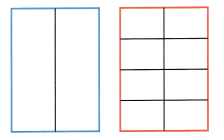The red rectangle has _________ equal parts than the blue rectangle.
(A) larger
(B) smaller
(C) fewer
Option (B)
Explanation:
The inverse relationship between the size of the fractional part and the number of equal parts in the whole when given the same size whole differs.
The greater the number of parts, the smaller the size of the parts and
the smaller the number of parts, the greater the size of the parts.

TAKE HOME ACTIVITY • Ask your child to show how he or she solved an exercise in the lesson.
Answer may vary from child to child.
Explanation:
By ding number of activities and learning the concept of pattern and blocks,
the students can able to explore the the equal parts.

### Texas Go Math Grade 2 Lesson 4.3 Homework and Practice Answer Key

Lesson Check

Question 6.
Which word completes the sentence?
The red circle has ___________ equal parts than the blue circle.(A) smaller
(B) fewer
(C) larger
Option(A)
Explanation:
The inverse relationship between the size of the fractional part and the number of equal parts in the whole when given the same size whole differs.
The greater the number of parts, the smaller the size of the parts and
the smaller the number of parts, the greater the size of the parts.

Question 7.
Three ribbons are the same size. The red ribbon is cut into fourths. The blue ribbon is cut into eighths. The green ribbon is cut into halves. Which ribbon has the largest equal parts?
(A) blue ribbon
(B) green ribbon
(C) red ribbon
Option(B)
Explanation:
The inverse relationship between the size of the fractional part and the number of equal parts in the whole when given the same size whole differs.
The greater the number of parts, the smaller the size of the parts and
the smaller the number of parts, the greater the size of the parts.

Question 8.
Three ropes are the same size. The orange rope is cut into halves. The brown rope is cut into eighths. The green rope is cut into fourths. Which rope has the smallest equal parts?
(A) orange rope
(B) green rope
(C) brown rope
Option(C)
Explanation:
The inverse relationship between the size of the fractional part and the number of equal parts in the whole when given the same size whole differs.
The greater the number of parts, the smaller the size of the parts and
the smaller the number of parts, the greater the size of the parts.

Question 9.
Sandy cuts three pies that are the same size. She cuts the apple pie into 6 equal slices, the peach pie into 8 equal slices, and the cherry pie into 4 equal slices. Which pie has the smallest slices?
(A) cherry pie
(B) peach pie
(C) apple pie